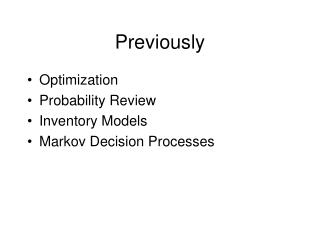DownloadDownload PresentationPreviously

# Previously

Download Presentation## Previously

- - - - - - - - - - - - - - - - - - - - - - - - - - - E N D - - - - - - - - - - - - - - - - - - - - - - - - - - -
##### Presentation Transcript

1. Previously • Optimization • Probability Review • Inventory Models • Markov Decision Processes

2. Agenda • Queues

3. number of servers: 1, 2, … distribution of the time between arrivals distribution of the processing time Queue Notation M / M / 1 M = ‘Markov’ exponential distribution D = ‘Deterministic’ constant G = ‘General’ other

4. service rate µ departures arrivals rate  queue servers c system Setup • W = E[T] time in systemWq = E[Tq] waiting time (time in queue) • L = E[N] #customers in systemLq = E[Nq] #customers in queue •  = /(cµ) utilization (fraction of time servers are busy)

5. Formulas • Simple • W = Wq + 1/µ • c average # of busy servers • L = Lq + c • Little’s Law: Lq =  Wq and L =  W • M/G/1 queue: ( 2 = variance of the service time )

6. Qualitatively • 1 means Lq • Lq increases with variability (of arrival / service times) • Lq decreases when pooling queues (a lot for M/M/1) ( or equivalently adding servers )

7. Simulation • What if not M/G/1? (ex. multiple servers) • What if qualitative results not enough?

8. Simulation • Online M/M/s http://www.usm.maine.edu/math/JPQ/ G/G/s http://staff.um.edu.mt/jskl1/simweb/sq1/sq1.html • Excel add-in (nothing for Excel 2008) From book (for M/M/s, fails for Excel 2007) QTP (fails on mac) http://www.business.ualberta.ca/aingolfsson/QTP/ ORMM bookqueue.xla at http://www.me.utexas.edu/~jensen/ORMM/frontpage/jensen.lib

9. ER Example (p508) Surgery c=3 µ=2/hr 12/hr 1/6 1/3 5/6 Diagnosis c=4 µ=4/hr 2/3 Other units

10. Networks of Queues (14.10) • Look at flow rates • Outflow =  when  < 1 • Time between arrivals not independent • formulas fail • Special case: all queues are M/M/s “Jackson Network” Lq just as if normal M/M/s queue